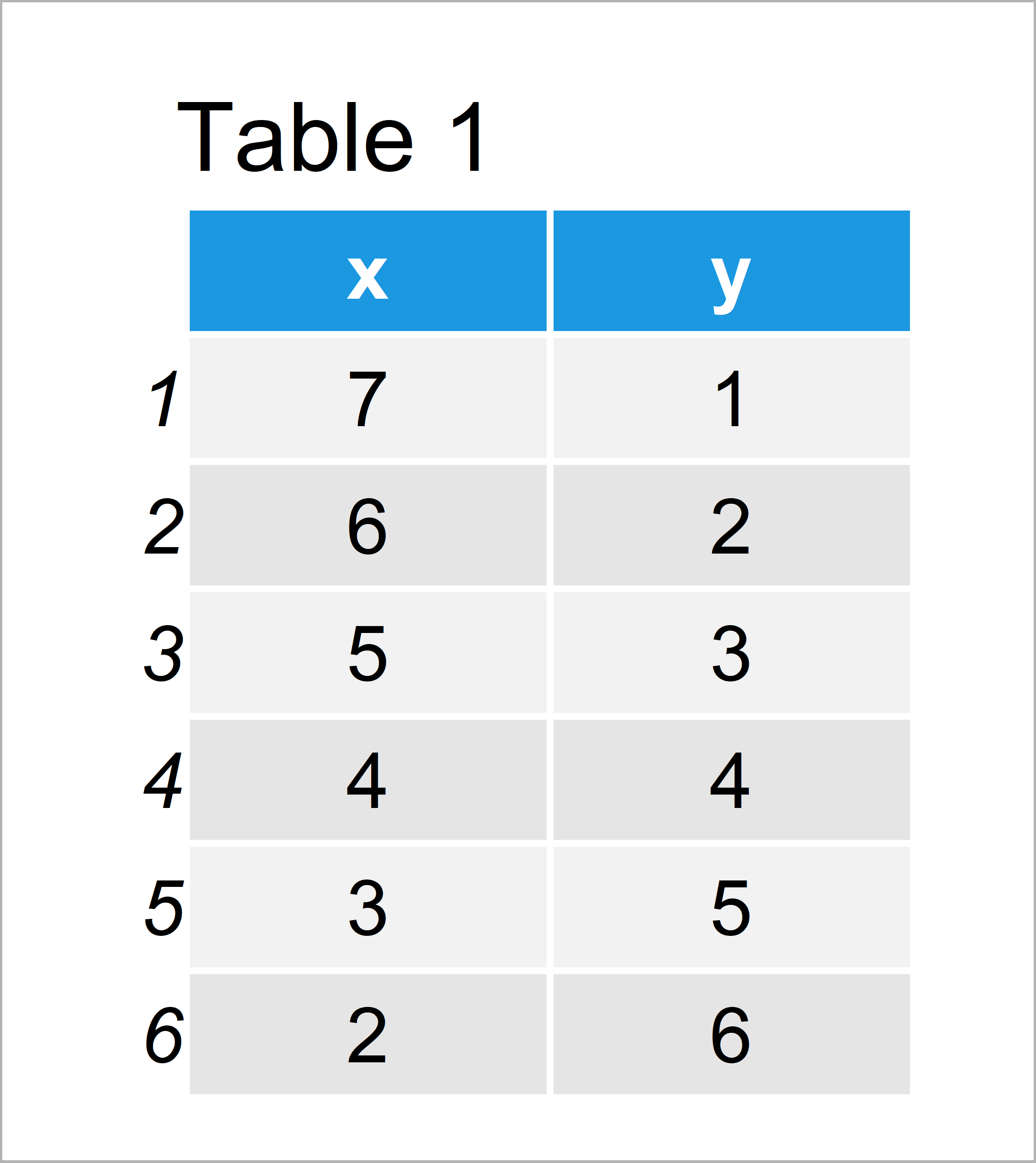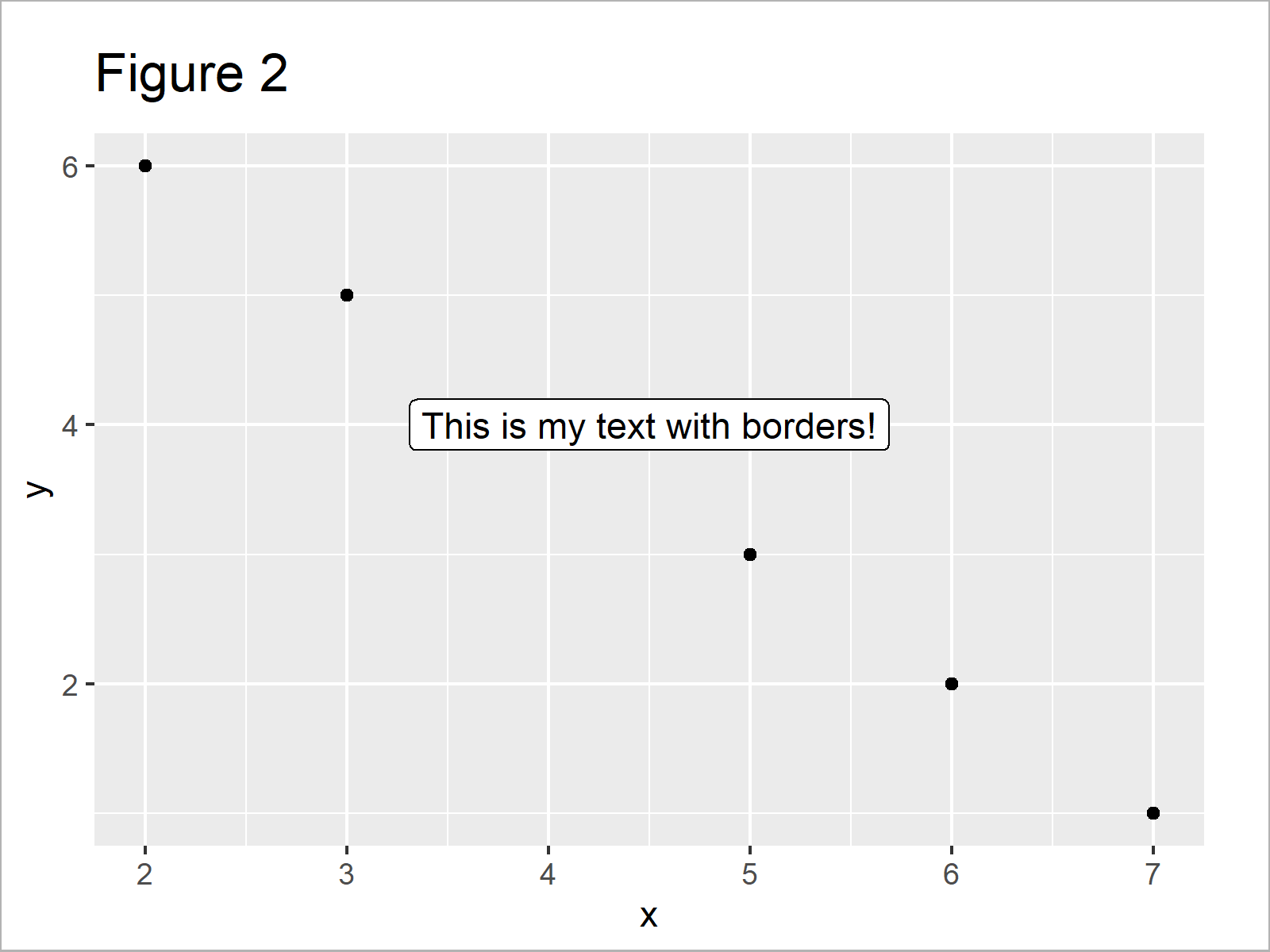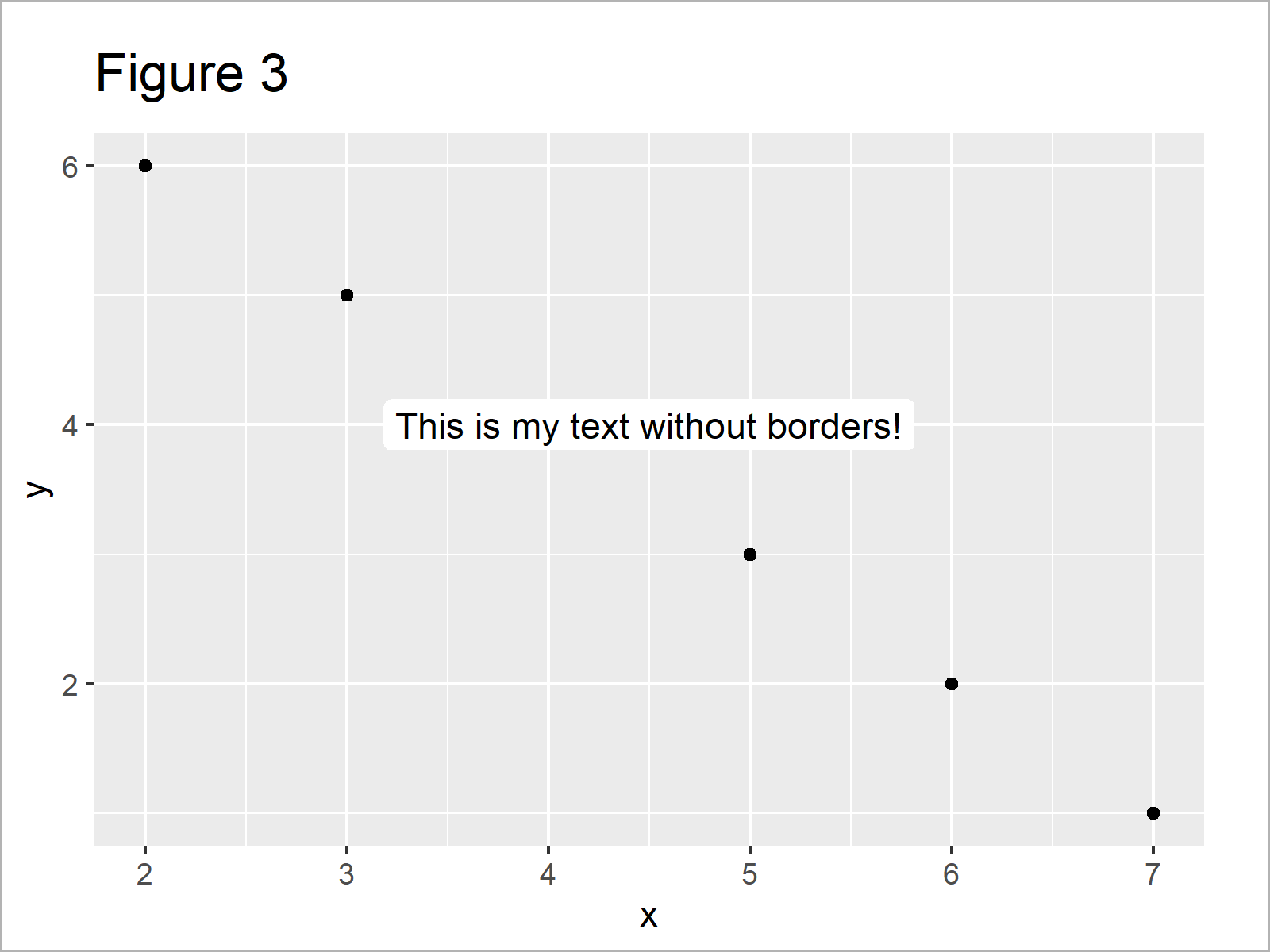# Remove Border of ggplot2 geom_label Text Annotation in R (Example)

In this R tutorial you’ll learn how to get rid of ggplot2 text annotation borders.

The article contains the following contents:

Here’s how to do it.

## Example Data, Packages & Default Plot

Let’s first create some example data in R.

```data <- data.frame(x = 7:2,         # Create example data
y = 1:6)
data                                # Print example data```Table 1 shows that the example data has six rows and two variables.

We also have to install and load the ggplot2 package, in order to use the corresponding functions:

```install.packages("ggplot2")         # Install & load ggplot2
library("ggplot2")```

Now, we can draw our data as shown below:

```ggp <- ggplot(data, aes(x, y)) +    # ggplot2 plot without text label
geom_point()
ggp                                 # Draw ggplot2 plot```As shown in Figure 1, the previous R programming code has plotted a ggplot2 scatterplot.

Now, we can add a text label to our plot using the geom_label function:

```ggp +                               # Adding text label to plot
geom_label(aes(x = 4.5,
y = 4,
label = "This is my text with borders!"))```The output of the previous syntax is visualized in Figure 2 – A ggplot2 scatterplot with text annotation within the plotting area of the plot.

As you can see, our text label has a black border. In the following example I’ll explain how to remove this border from our text box.

## Example: Remove Border of ggplot2 Text Label Using label.size

The syntax below demonstrates how to get rid of the border around our geom_label text box.

For this task, we have to specify the label.size to be equal to NA (i.e. Not Available):

```ggp +                               # Remove text label border using label.size
geom_label(aes(x = 4.5,
y = 4,
label = "This is my text without borders!"),
label.size = NA)```In Figure 3 you can see that we have managed to create a new graphic that contains a text label without borders with the previous R syntax.

## Video & Further Resources

Do you need more information on the R code of this post? Then I recommend watching the following video of my YouTube channel. In the video, I show the R programming syntax of this tutorial in the R programming language.

Please accept YouTube cookies to play this video. By accepting you will be accessing content from YouTube, a service provided by an external third party.If you accept this notice, your choice will be saved and the page will refresh.

In addition, you may want to have a look at some of the related posts of my homepage. You can find some articles about ggplot2 here.